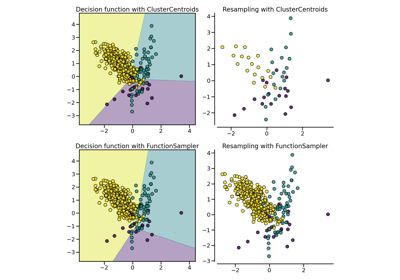# imblearn.under_sampling.ClusterCentroids¶

class imblearn.under_sampling.ClusterCentroids(*, sampling_strategy='auto', random_state=None, estimator=None, voting='auto', n_jobs='deprecated')[source]

Undersample by generating centroids based on clustering methods.

Method that under samples the majority class by replacing a cluster of majority samples by the cluster centroid of a KMeans algorithm. This algorithm keeps N majority samples by fitting the KMeans algorithm with N cluster to the majority class and using the coordinates of the N cluster centroids as the new majority samples.

Read more in the User Guide.

Parameters
sampling_strategyfloat, str, dict, callable, default=’auto’

Sampling information to sample the data set.

• When float, it corresponds to the desired ratio of the number of samples in the minority class over the number of samples in the majority class after resampling. Therefore, the ratio is expressed aswhereis the number of samples in the minority class andis the number of samples in the majority class after resampling.

Warning

float is only available for binary classification. An error is raised for multi-class classification.

• When str, specify the class targeted by the resampling. The number of samples in the different classes will be equalized. Possible choices are:

'majority': resample only the majority class;

'not minority': resample all classes but the minority class;

'not majority': resample all classes but the majority class;

'all': resample all classes;

'auto': equivalent to 'not minority'.

• When dict, the keys correspond to the targeted classes. The values correspond to the desired number of samples for each targeted class.

• When callable, function taking y and returns a dict. The keys correspond to the targeted classes. The values correspond to the desired number of samples for each class.

random_stateint, RandomState instance, default=None

Control the randomization of the algorithm.

• If int, random_state is the seed used by the random number generator;

• If RandomState instance, random_state is the random number generator;

• If None, the random number generator is the RandomState instance used by np.random.

estimatorobject, default=KMeans()

Pass a sklearn.cluster.KMeans estimator.

voting{“hard”, “soft”, “auto”}, default=’auto’

Voting strategy to generate the new samples:

• If 'hard', the nearest-neighbors of the centroids found using the clustering algorithm will be used.

• If 'soft', the centroids found by the clustering algorithm will be used.

• If 'auto', if the input is sparse, it will default on 'hard' otherwise, 'soft' will be used.

New in version 0.3.0.

n_jobsint, default=None

Number of CPU cores used during the cross-validation loop. None means 1 unless in a joblib.parallel_backend context. -1 means using all processors. See Glossary for more details.

Deprecated since version 0.7: n_jobs was deprecated in 0.7 and will be removed in 0.9.

EditedNearestNeighbours

Under-sampling by editing samples.

CondensedNearestNeighbour

Under-sampling by condensing samples.

Notes

Supports multi-class resampling by sampling each class independently.

Examples

>>> from collections import Counter
>>> from sklearn.datasets import make_classification
>>> from imblearn.under_sampling import ClusterCentroids
>>> X, y = make_classification(n_classes=2, class_sep=2,
... weights=[0.1, 0.9], n_informative=3, n_redundant=1, flip_y=0,
... n_features=20, n_clusters_per_class=1, n_samples=1000, random_state=10)
>>> print('Original dataset shape %s' % Counter(y))
Original dataset shape Counter({1: 900, 0: 100})
>>> cc = ClusterCentroids(random_state=42)
>>> X_res, y_res = cc.fit_resample(X, y)
>>> print('Resampled dataset shape %s' % Counter(y_res))
...
Resampled dataset shape Counter({...})

__init__(self, *, sampling_strategy='auto', random_state=None, estimator=None, voting='auto', n_jobs='deprecated')[source]

Initialize self. See help(type(self)) for accurate signature.

## Examples using imblearn.under_sampling.ClusterCentroids¶